# Interesting CSS math functions

2021-08-26 15:47:07

## Preface

Hello everyone , Here is CSS and WebGL Magic ——alphardex.

I've been playing three.js , Touched a lot of mathematical functions , Used them to create a lot of special effects . So I thought ： Can it be in CSS These mathematical functions are also used in , But found CSS Not yet , It is said that the future new specifications will be incorporated into , I guess it will take a long time .

However , We can do it with a few tricks , To create something of your own CSS Mathematical functions , So as to achieve some interesting animation effects .

Let's get started ！

## CSS Mathematical functions

Be careful ： The following functions use native CSS It can also be realized , Here we use SCSS Function is just for convenience of encapsulation , If encapsulated, it is more convenient to call

### The absolute value

Is the absolute value positive or positive , Negative becomes positive

Can create 2 Number , One of them is the opposite of the other , Compare their maximum values , You can get the absolute value of this number

``````@function abs(\$v) {
@return max(#{\$v}, calc(-1 * #{\$v}));
}
Copy code ``````

### Median

Original number minus 1 And multiply by half

``````@function middle(\$v) {
@return calc(0.5 * (#{\$v} - 1));
}
Copy code ``````

### The distance between two points on the number axis

The distance between two points on the number axis is the absolute value of the difference between the numbers represented by two points , With the above absolute value formula, you can write it directly

``````@function dist-1d(\$v1, \$v2) {
\$v-delta: calc(#{\$v1} - #{\$v2});
@return #{abs(\$v-delta)};
}
Copy code ``````

### Trigonometric functions

In fact, the author will not realize ~ But I've seen it before Good friends chokcoco An article from Wrote about how to CSS Trigonometric function in , Thank you

``````@function fact(\$number) {
\$value: 1;
@if \$number>0 {
@for \$i from 1 through \$number {
\$value: \$value * \$i;
}
}
@return \$value;
}

@function pow(\$number, \$exp) {
\$value: 1;
@if \$exp>0 {
@for \$i from 1 through \$exp {
\$value: \$value * \$number;
}
} @else if \$exp < 0 {
@for \$i from 1 through -\$exp {
\$value: \$value / \$number;
}
}
@return \$value;
}

\$unit: unit(\$angle);
\$unitless: \$angle / (\$angle * 0 + 1);
@if \$unit==deg {
\$unitless: \$unitless / 180 * pi();
}
@return \$unitless;
}

@function pi() {
@return 3.14159265359;
}

@function sin(\$angle) {
\$sin: 0;
// Iterate a bunch of times.
@for \$i from 0 through 20 {
\$sin: \$sin + pow(-1, \$i) * pow(\$angle, (2 * \$i + 1)) / fact(2 * \$i + 1);
}
@return \$sin;
}

@function cos(\$angle) {
\$cos: 0;
// Iterate a bunch of times.
@for \$i from 0 through 20 {
\$cos: \$cos + pow(-1, \$i) * pow(\$angle, 2 * \$i) / fact(2 * \$i);
}
@return \$cos;
}

@function tan(\$angle) {
@return sin(\$angle) / cos(\$angle);
}
Copy code ``````

## Example

The following animation effects demonstrate the role of the above mathematical functions

### One dimensional interlaced animation

#### The initial state

Create a row of elements , Fill... With internal shadows , Prepare our mathematical functions

``````<div class="list">
<div class="list-item"></div>
...( Omit here 14 individual  list-item)
<div class="list-item"></div>
</div>
Copy code ``````
``````body {
display: flex;
justify-content: center;
align-items: center;
min-height: 100vh;
margin: 0;
background: #222;
}

:root {
--blue-color-1: #6ee1f5;
}

( Copy and paste all the above mathematical formulas here )

.list {
--n: 16;

display: flex;
flex-wrap: wrap;
justify-content: space-evenly;

&-item {
--p: 2vw;
--gap: 1vw;
--bg: var(--blue-color-1);

@for \$i from 1 through 16 {
&:nth-child(#{\$i}) {
--i: #{\$i};
}
}

margin: var(--gap);
box-shadow: inset 0 0 0 var(--p) var(--bg);
}
}
Copy code ``````#### Apply animation

This is used here. 2 An animation ：grow Responsible for scaling out elements ;melt be responsible for “ melt ” Elements （ That is, the diffusion radius of eliminating shadows ）

``````<div class="list grow-melt">
<div class="list-item"></div>
...( Omit here 14 individual  list-item)
<div class="list-item"></div>
</div>
Copy code ``````
``````.list {
&.grow-melt {
.list-item {
--t: 2s;

animation-name: grow, melt;
animation-duration: var(--t);
animation-iteration-count: infinite;
}
}
}

@keyframes grow {
0% {
transform: scale(0);
}

50%,
100% {
transform: scale(1);
}
}

@keyframes melt {
0%,
50% {
box-shadow: inset 0 0 0 var(--p) var(--bg);
}

100% {
box-shadow: inset 0 0 0 0 var(--bg);
}
}
Copy code ``````#### Interlace animation

1. Calculate the median of the element subscript
2. Calculate each element id The distance to this median
3. Calculate the scale according to the distance
4. Calculate... According to the proportion delay
``````<div class="list grow-melt middle-stagger">
<div class="list-item"></div>
...( Omit here 14 individual  list-item)
<div class="list-item"></div>
</div>
Copy code ``````
``````.list {
&.middle-stagger {
.list-item {
--m: #{middle(var(--n))}; //  Median , Here is 7.5
--i-m-dist: #{dist-1d(var(--i), var(--m))}; //  Calculate each id The distance to the median
--ratio: calc(var(--i-m-dist) / var(--m)); //  Calculate the scale according to the distance
--delay: calc(var(--ratio) * var(--t)); //  Calculate... According to the proportion delay
--n-delay: calc((var(--ratio) - 2) * var(--t)); //  negative delay Indicates that the animation starts in advance

animation-delay: var(--n-delay);
}
}
}
Copy code ``````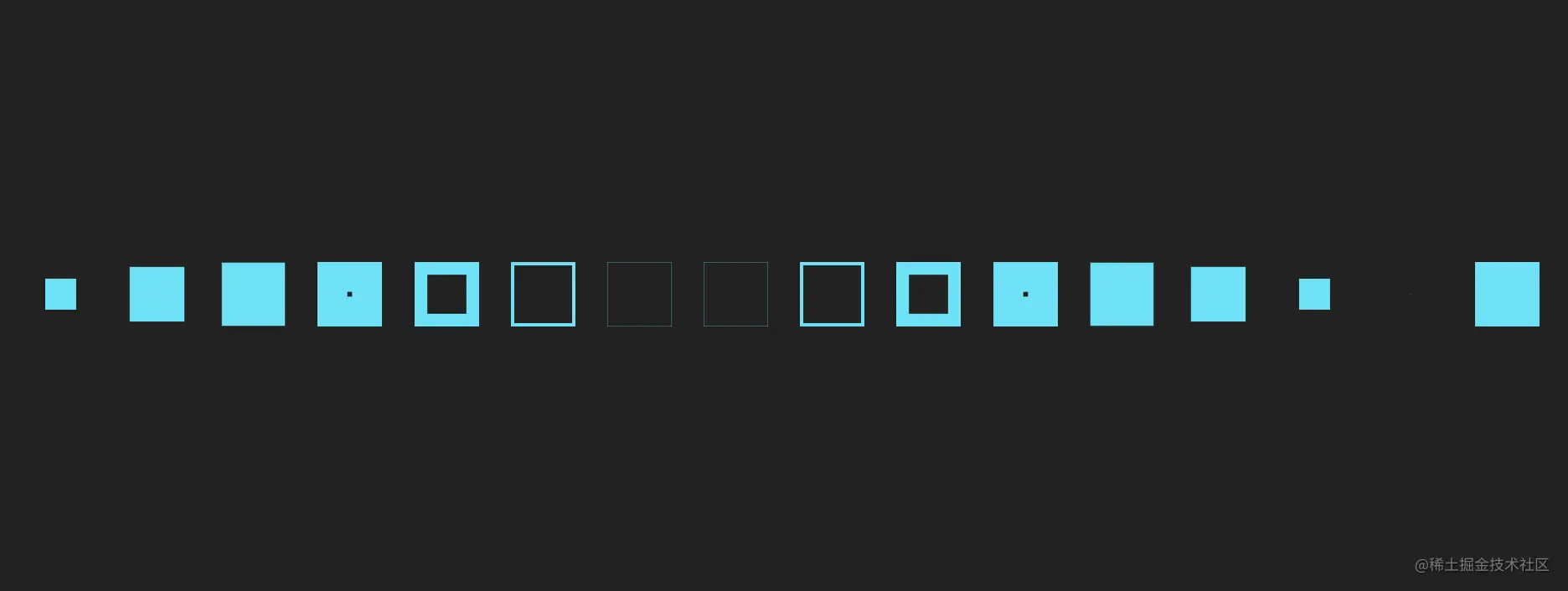### 2D interlaced animation

#### The initial state

How to turn one-dimensional into two-dimensional ？ Apply grid system

``````<div class="grid">
<div class="grid-item"></div>
...( Omit here 62 individual  grid-item)
<div class="grid-item"></div>
</div>
Copy code ``````
``````.grid {
\$row: 8;
\$col: 8;
--row: #{\$row};
--col: #{\$col};
--gap: 0.25vw;

display: grid;
gap: var(--gap);
grid-template-rows: repeat(var(--row), 1fr);
grid-template-columns: repeat(var(--col), 1fr);

&-item {
--p: 2vw;
--bg: var(--blue-color-1);

@for \$y from 1 through \$row {
@for \$x from 1 through \$col {
\$k: \$col * (\$y - 1) + \$x;
&:nth-child(#{\$k}) {
--x: #{\$x};
--y: #{\$y};
}
}
}

box-shadow: inset 0 0 0 var(--p) var(--bg);
}
}
Copy code ``````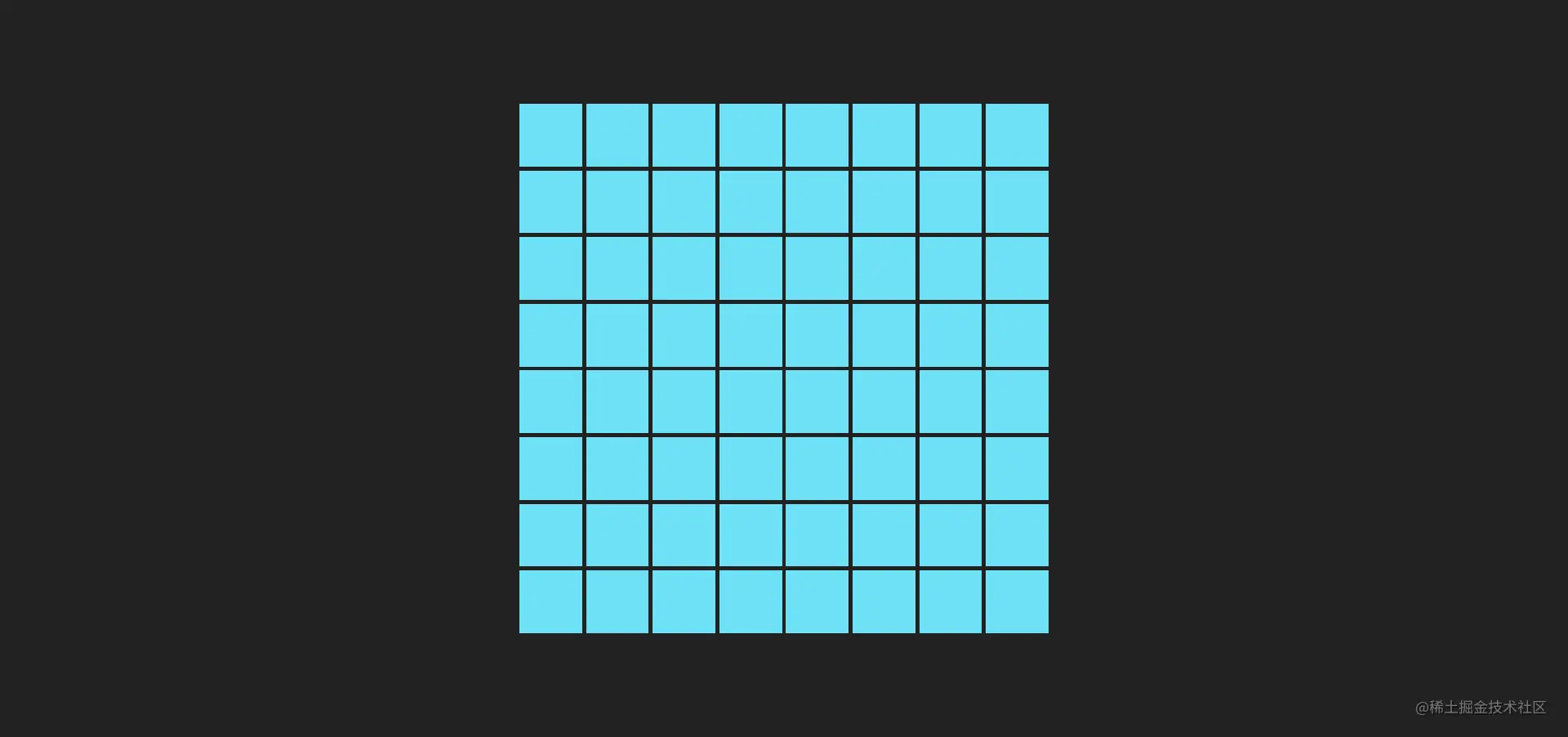#### Apply animation

As like as two peas

``````<div class="grid grow-melt">
<div class="grid-item"></div>
...( Omit here 62 individual  grid-item)
<div class="grid-item"></div>
</div>
Copy code ``````
``````.grid {
&.grow-melt {
.grid-item {
--t: 2s;

animation-name: grow, melt;
animation-duration: var(--t);
animation-iteration-count: infinite;
}
}
}
Copy code ``````#### Interlace animation

1. Calculate the median of grid rows and columns
2. Computing grid xy The distance from the coordinates to the median and sum
3. Calculate the scale according to the distance
4. Calculate... According to the proportion delay
``````<div class="grid grow-melt middle-stagger">
<div class="grid-item"></div>
...( Omit here 62 individual  grid-item)
<div class="grid-item"></div>
</div>
Copy code ``````
``````.grid {
&.middle-stagger {
.grid-item {
--m: #{middle(var(--col))}; //  Median , Here is 7.5
--x-m-dist: #{dist-1d(var(--x), var(--m))}; //  Calculation x The distance from the coordinates to the median
--y-m-dist: #{dist-1d(var(--y), var(--m))}; //  Calculation y The distance from the coordinates to the median
--dist-sum: calc(var(--x-m-dist) + var(--y-m-dist)); //  The sum of distances
--ratio: calc(var(--dist-sum) / var(--m)); //  Calculate scale based on distance and
--delay: calc(var(--ratio) * var(--t) * 0.5); //  Calculate... According to the proportion delay
--n-delay: calc(
(var(--ratio) - 2) * var(--t) * 0.5
); //  negative delay Indicates that the animation starts in advance

animation-delay: var(--n-delay);
}
}
}
Copy code ``````#### Another animation

You can change the animation shuffle（ shuttle ）, Will produce another strange effect

``````<div class="grid shuffle middle-stagger">
<div class="grid-item"></div>
...( Omit here 254 individual  grid-item )
<div class="grid-item"></div>
</div>
Copy code ``````
``````.grid {
\$row: 16;
\$col: 16;
--row: #{\$row};
--col: #{\$col};
--gap: 0.25vw;

&-item {
--p: 1vw;

transform-origin: bottom;
transform: scaleY(0.1);
}

&.shuffle {
.grid-item {
--t: 2s;

animation: shuffle var(--t) infinite ease-in-out alternate;
}
}
}

@keyframes shuffle {
0% {
transform: scaleY(0.1);
}

50% {
transform: scaleY(1);
transform-origin: bottom;
}

50.01% {
transform-origin: top;
}

100% {
transform-origin: top;
transform: scaleY(0.1);
}
}
Copy code ``````### Cosine wave animation

#### The initial state

establish 7 A different color （ The rainbow color is directly selected here ） list , Each list has 40 Sub elements , Each child element is a dot

Let this 7 A list is arranged on a line , And z The distance on the axis is staggered , Set up the basic delay

``````<div class="lists">
<div class="list">
<div class="list-item"></div>
...( Omit here 39 individual  list-item)
</div>
...( Omit here 6 individual  list)
</div>
Copy code ``````
``````.lists {
\$list-count: 7;
\$colors: red, orange, yellow, green, cyan, blue, purple;

position: relative;
width: 34vw;
height: 2vw;
transform-style: preserve-3d;
perspective: 800px;

.list {
position: absolute;
top: 0;
left: 0;
display: flex;
transform: translateZ(var(--z));

@for \$i from 1 through \$list-count {
&:nth-child(#{\$i}) {
--bg: #{nth(\$colors, \$i)};
--z: #{\$i * -1vw};
--basic-delay-ratio: #{\$i / \$list-count};
}
}

&-item {
--w: 0.6vw;
--gap: 0.15vw;

width: var(--w);
height: var(--w);
margin: var(--gap);
background: var(--bg);
}
}
}
Copy code ``````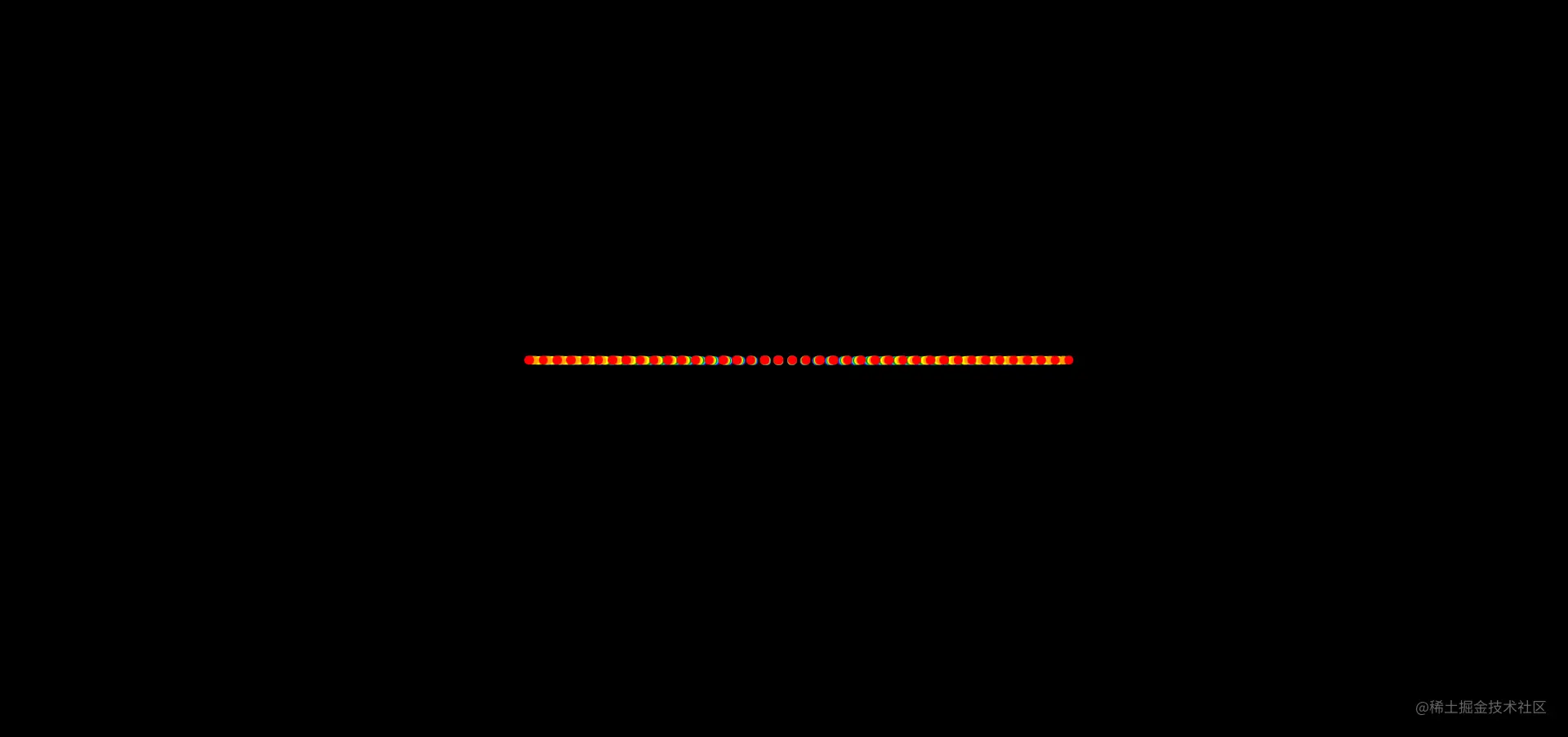#### Cosine permutation

Using the trigonometric function formula above , Let these dots be arranged in the shape of a part of the cosine

``````.lists {
.list {
&-item {
\$item-count: 40;
\$offset: pi() * 0.5;
--wave-length: 21vw;

@for \$i from 1 through \$item-count {
&:nth-child(#{\$i}) {
--i: #{\$i};
\$ratio: (\$i - 1) / (\$item-count - 1);
\$angle-unit: pi() * \$ratio;
\$wave: cos(\$angle-unit + \$offset);
--single-wave-length: calc(#{\$wave} * var(--wave-length));
--n-single-wave-length: calc(var(--single-wave-length) * -1);
}
}

transform: translateY(var(--n-single-wave-length));
}
}
}
Copy code ``````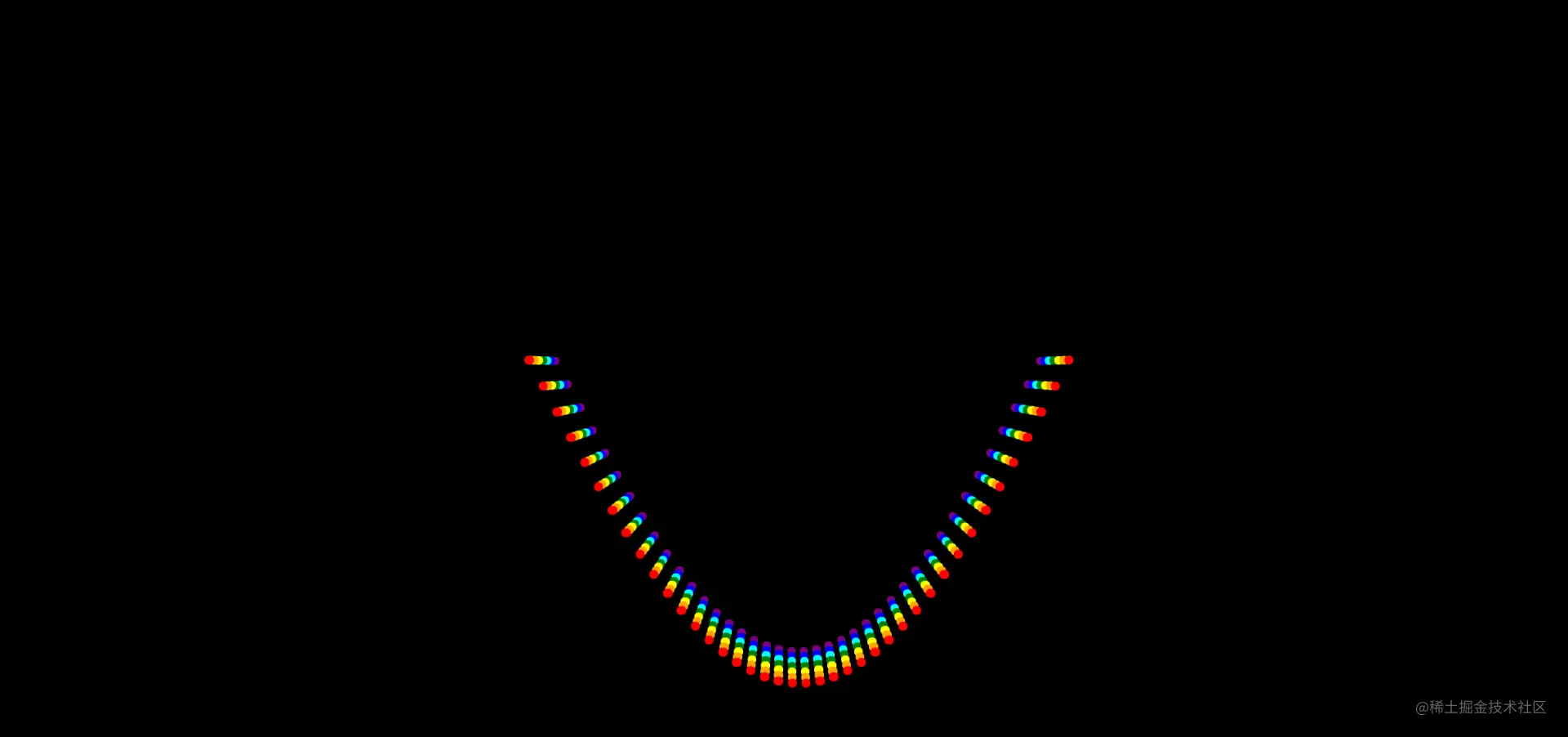#### Wave animation

Apply up and down translation animation to each small circle point , The translation distance is the cosine wave distance

``````.lists {
.list {
&-item {
--t: 2s;

animation: wave var(--t) infinite ease-in-out alternate;
}
}
}

@keyframes wave {
from {
transform: translateY(var(--n-single-wave-length));
}

to {
transform: translateY(var(--single-wave-length));
}
}
Copy code ``````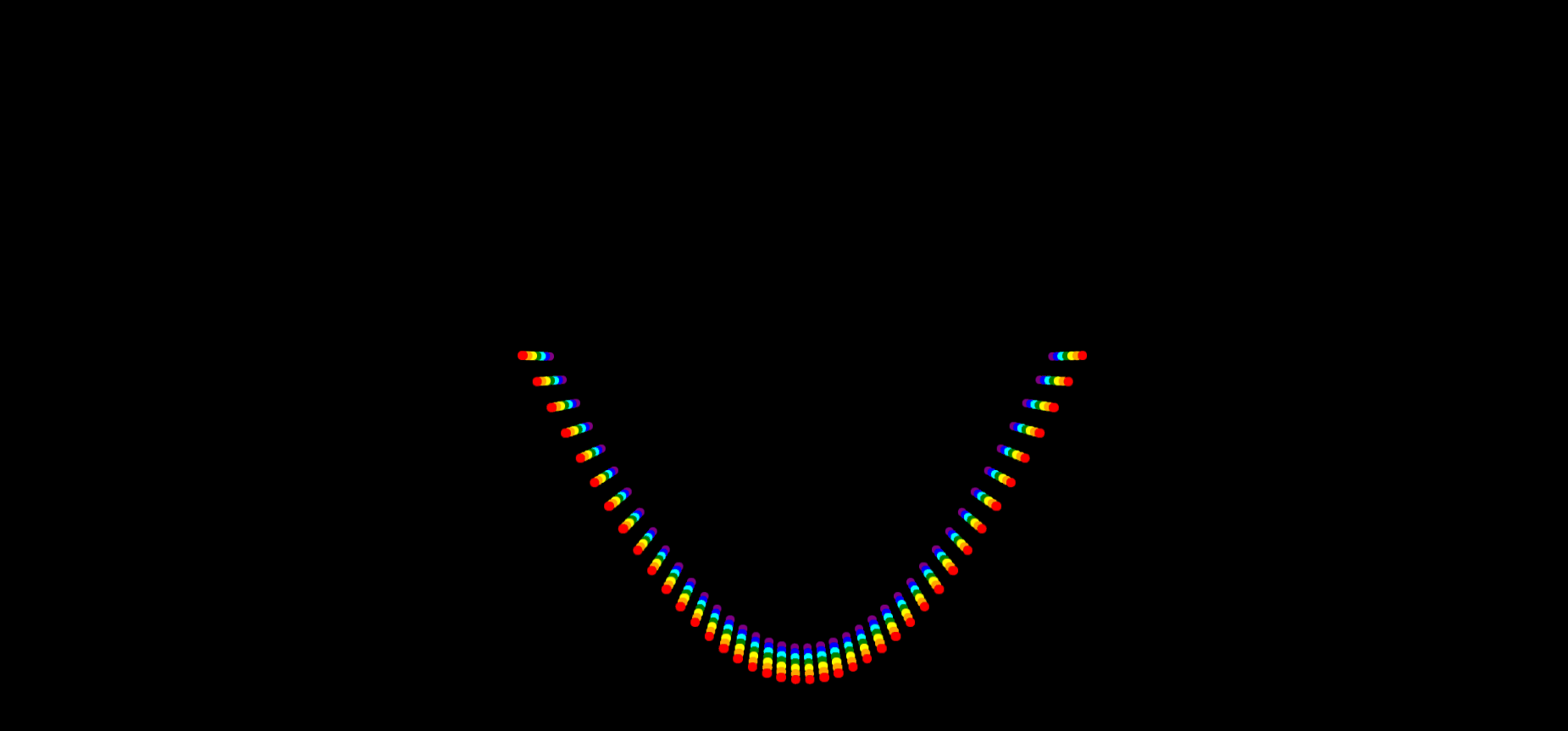#### Interlace animation

Follow the above routine , The calculation starts in the middle delay, Then apply it to the animation

``````.lists {
.list {
&-item {
--n: #{\$item-count + 1};
--m: #{middle(var(--n))};
--i-m-dist: #{dist-1d(var(--i), var(--m))};
--ratio: calc(var(--i-m-dist) / var(--m));
--square: calc(var(--ratio) * var(--ratio));
--delay: calc(
calc(var(--square) + var(--basic-delay-ratio) + 1) * var(--t)
);
--n-delay: calc(var(--delay) * -1);

animation-delay: var(--n-delay);
}
}
}
Copy code ``````## Last

CSS Mathematical functions can achieve far more special effects than this , I hope this article can inspire you to create special effects ~